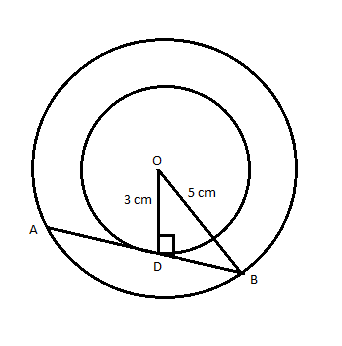QUESTION

# Two concentric circles are of radii 5 cm and 3 cm. Find the length of chord of the larger circle which touches the smaller circle.

Hint: In this question first draw the diagram it will give us a clear picture of what we have to find out. Then use the property that the radius is perpendicular to a chord and it divides the chord into two equal parts. So, use this property to reach the solution of the given problem.

Let the radius of the bigger circle be $R$and the radius of the smaller circle is $r$.
It is given that $R = 5{\text{ cm}}$and $r = 3{\text{ cm}}$

The diagram of the two concentric circles is given below:Here AB is the larger chord whose length we have to find.
By using the property that the radius is perpendicular to a chord and it divides the chord into two equal parts, we have $AD = BD$and $OD \bot AB$
Therefore, $\Delta ODB$is a right-angled triangle.
As $\Delta ODB$is a right-angled, we have
$\Rightarrow O{D^2} + B{D^2} = O{B^2} \\ \Rightarrow {\left( 3 \right)^2} + B{D^2} = {\left( 5 \right)^2} \\ \Rightarrow B{D^2} = {\left( 5 \right)^2} - {\left( 3 \right)^2} \\ \Rightarrow B{D^2} = 25 - 9 \\ \Rightarrow B{D^2} = 16 \\ \therefore BD = 4{\text{ cm}} \\$

So, AD = BD = 4 cm

Hence, AB = AD + BD = 4 + 4 = 8 cm.

Thus, the length of the larger chord is 8 cm.

Note: Here we have not considered the negative of BD because the length of the chord is always positive. Concentric circles are circles with a common centre. They fit inside each other and are the same distance apart all the way around.